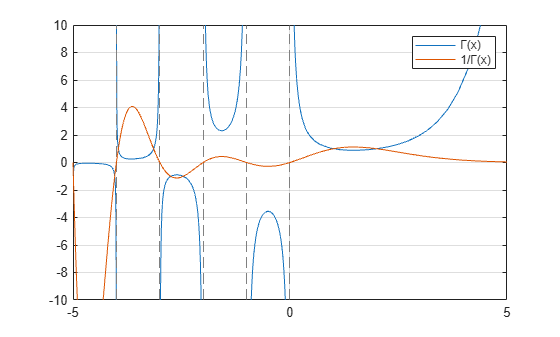gamma

Gamma function

Description

example

Y = gamma(X) returns the gamma function evaluated at the elements of X.

Examples

collapse all

Evaluate the gamma function with a scalar and a vector.

Evaluate $\Gamma \left(0.5\right)$, which is equal to $\sqrt{\pi }$.

y = gamma(0.5)
y = 1.7725

Evaluate several values of the gamma function between [-3.5 3.5].

x = -3.5:3.5;
y = gamma(x)
y = 1×8

0.2701   -0.9453    2.3633   -3.5449    1.7725    0.8862    1.3293    3.3234

Plot the gamma function and its reciprocal.

Use fplot to plot the gamma function and its reciprocal. The gamma function increases quickly for positive arguments and has simple poles at all negative integer arguments (as well as 0). The function does not have any zeros. Conversely, the reciprocal gamma function has zeros at all negative integer arguments (as well as 0).

fplot(@gamma)
hold on
fplot(@(x) 1./gamma(x))
ylim([-10 10])
legend('\Gamma(x)','1/\Gamma(x)')
hold off
grid onInput Arguments

collapse all

Input array, specified as a scalar, vector, matrix, or multidimensional array. The elements of X must be real.

Data Types: single | double

collapse all

Gamma Function

The gamma function is defined for real x > 0 by the integral:

$\Gamma \left(x\right)={\int }_{0}^{\infty }{e}^{-t}{t}^{x-1}dt$

The gamma function interpolates the factorial function. For integer n:

gamma(n+1) = factorial(n) = prod(1:n)

The domain of the gamma function extends to negative real numbers by analytic continuation, with simple poles at the negative integers. This extension arises from repeated application of the recursion relation

$\Gamma \left(n-1\right)=\frac{\Gamma \left(n\right)}{n-1}\text{\hspace{0.17em}}.$

Algorithms

The computation of gamma is based on algorithms outlined in .

 Cody, J., An Overview of Software Development for Special Functions, Lecture Notes in Mathematics, 506, Numerical Analysis Dundee, G. A. Watson (ed.), Springer Verlag, Berlin, 1976.

 Abramowitz, M. and I.A. Stegun, Handbook of Mathematical Functions, National Bureau of Standards, Applied Math. Series #55, Dover Publications, 1965, sec. 6.5.